The SEVERITY Procedure

Parameter Estimation Method

Subsections:

If you do not specify a custom objective function by specifying programming statements and the OBJECTIVE= option in the PROC SEVERITY statement, then PROC SEVERITY uses the maximum likelihood (ML) method to estimate the parameters of each model. A nonlinear optimization process is used to maximize the log of the likelihood function. If you specify a custom objective function, then PROC SEVERITY uses a nonlinear optimization algorithm to estimate the parameters of each model that minimize the value of your specified objective function. For more information, see the section Custom Objective Functions.

Likelihood Function

Let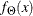and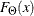denote the PDF and CDF, respectively, evaluated at x for a set of parameter values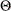. Let Y denote the random response variable, and let y denote its value recorded in an observation in the input data set. Let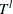and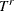denote the random variables for the left-truncation and right-truncation threshold, respectively, and let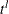and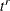denote their values for an observation, respectively. If there is no left-truncation, then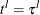, where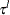is the smallest value in the support of the distribution; so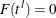. If there is no right-truncation, then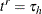, where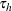is the largest value in the support of the distribution; so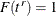. Let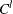and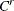denote the random variables for the left-censoring and right-censoring limit, respectively, and let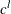and
denote their values for an observation, respectively. If there is no left-censoring, then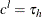; so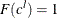. If there is no right-censoring, then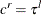; so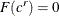.

The set of input observations can be categorized into the following four subsets within each BY group:

• E is the set of uncensored and untruncated observations. The likelihood of an observation in E is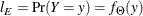•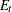is the set of uncensored observations that are truncated. The likelihood of an observation inis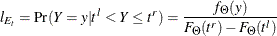• C is the set of censored observations that are not truncated. The likelihood of an observation C is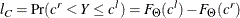•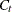is the set of censored observations that are truncated. The likelihood of an observationis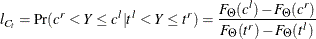Note that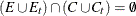. Also, the setsandare empty when you do not specify truncation, and the sets C andare empty when you do not specify censoring.

Given this, the likelihood of the data L is as follows: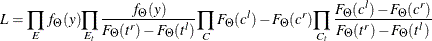The maximum likelihood procedure used by PROC SEVERITY finds an optimal set of parameter valuesthat maximizes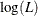subject to the boundary constraints on parameter values. For a distribution dist, you can specify such boundary constraints by using the dist_LOWERBOUNDS and dist_UPPERBOUNDS subroutines. For more information, see the section Defining a Severity Distribution Model with the FCMP Procedure. Some aspects of the optimization process can be controlled by using the NLOPTIONS statement.

Probability of Observability and Likelihood

If you specify the probability of observability for the left-truncation, then PROC SEVERITY uses a modified likelihood function for each truncated observation. If the probability of observability is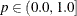, then for each left-truncated observation with truncation threshold, there exist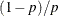observations with a response variable value less than or equal to. Each such observation has a probability of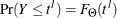. The right-truncation and censoring information does not apply to these added observations. Thus, following the notation of the section Likelihood Function, the likelihood of the data is as follows: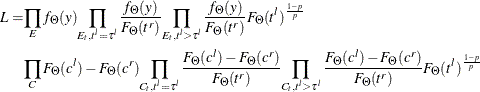Note that the likelihood of the observations that are not left-truncated (observations in sets E and C, and observations in setsandfor which) is not affected.

If you specify a custom objective function, then PROC SEVERITY accounts for the probability of observability only while computing the empirical distribution function estimate. The parameter estimates are affected only by your custom objective function.

Estimating Covariance and Standard Errors

PROC SEVERITY computes an estimate of the covariance matrix of the parameters by using the asymptotic theory of the maximum likelihood estimators (MLE). If N denotes the number of observations used for estimating a parameter vector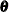, then the theory states that as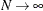, the distribution of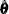, the estimate of, converges to a normal distribution with meanand covariance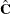such that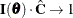, where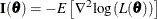is the information matrix for the likelihood of the data,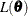. The covariance estimate is obtained by using the inverse of the information matrix.

In particular, if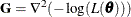denotes the Hessian matrix of the negative of log likelihood, then the covariance estimate is computed as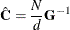where d is a denominator that is determined by the VARDEF= option. If VARDEF=N, then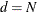, which yields the asymptotic covariance estimate. If VARDEF=DF, then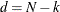, where k is number of parameters (the model’s degrees of freedom). The VARDEF=DF option is the default, because it attempts to correct the potential bias introduced by the finite sample.

The standard error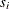of the parameter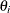is computed as the square root of the ith diagonal element of the estimated covariance matrix; that is,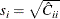.

If you specify a custom objective function, then the covariance matrix of the parameters is still computed by inverting the information matrix, except that the Hessian matrixis computed as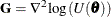, where U denotes your custom objective function that is minimized by the optimizer.

Covariance and standard error estimates might not be available if the Hessian matrix is found to be singular at the end of the optimization process. This can especially happen if the optimization process stops without converging.